# 吴恩达机器学习笔记-支持向量机

## 优化目标

h_\theta(x) = \frac{1}{1+e^{-\theta^Tx}}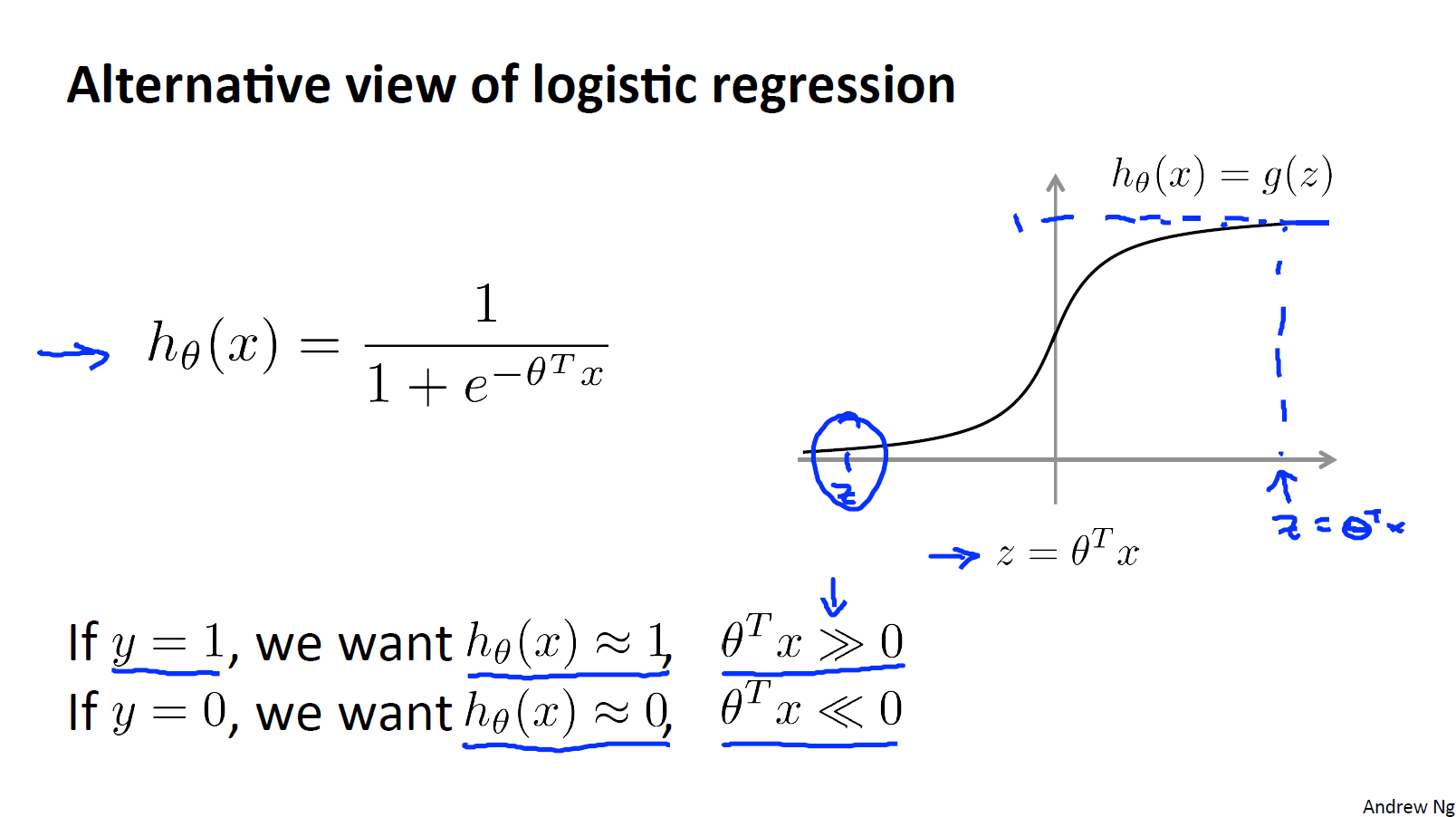-(ylogh_\theta(x) + (1-y)log(1-h_\theta(x))) = -ylog\frac{1}{1+e^{-\theta^Tx}} - (1-y)log(1-log\frac{1}{1+e^{-\theta^Tx}})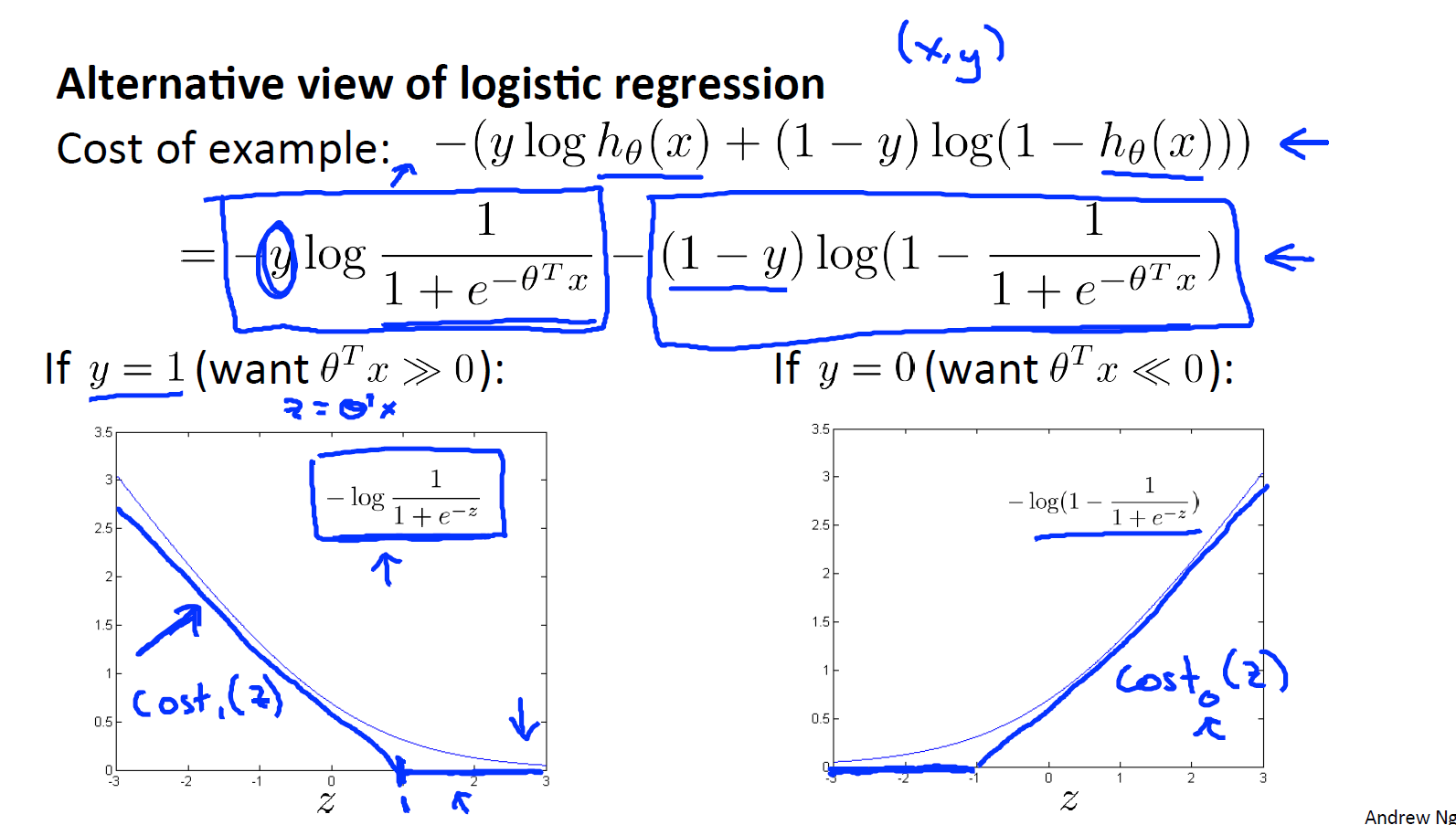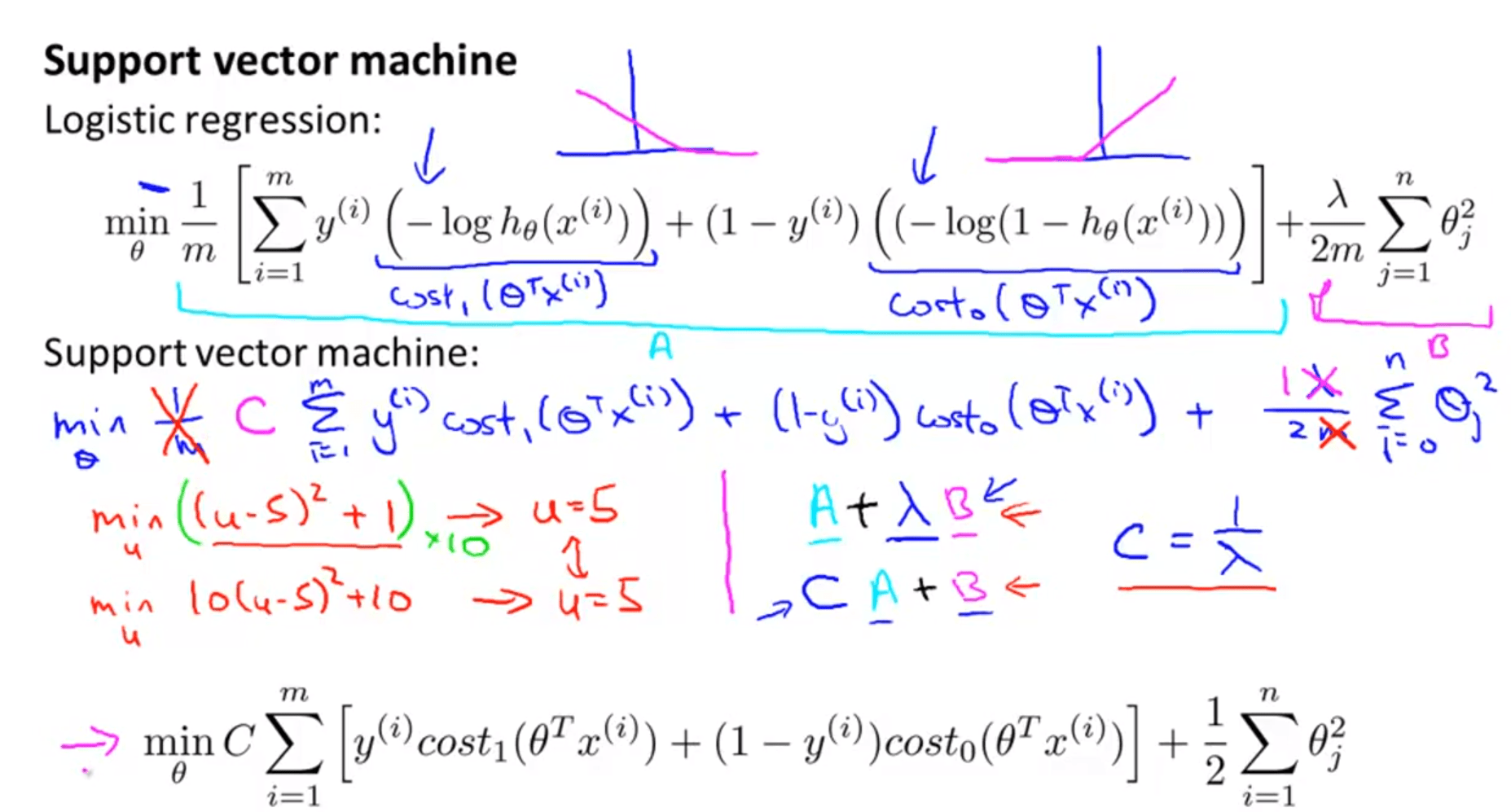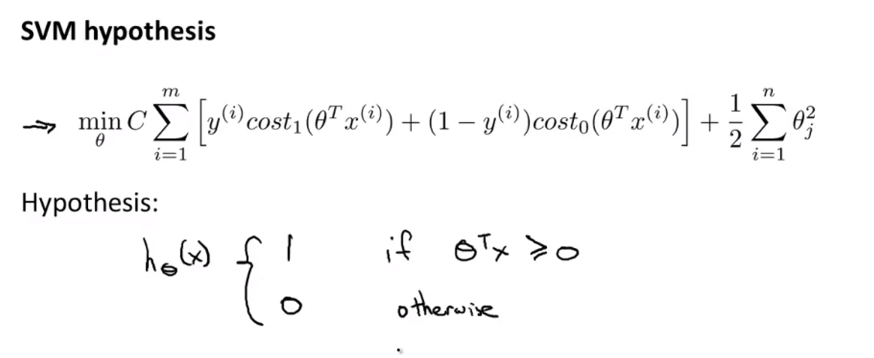## 直观上对大间隔的理解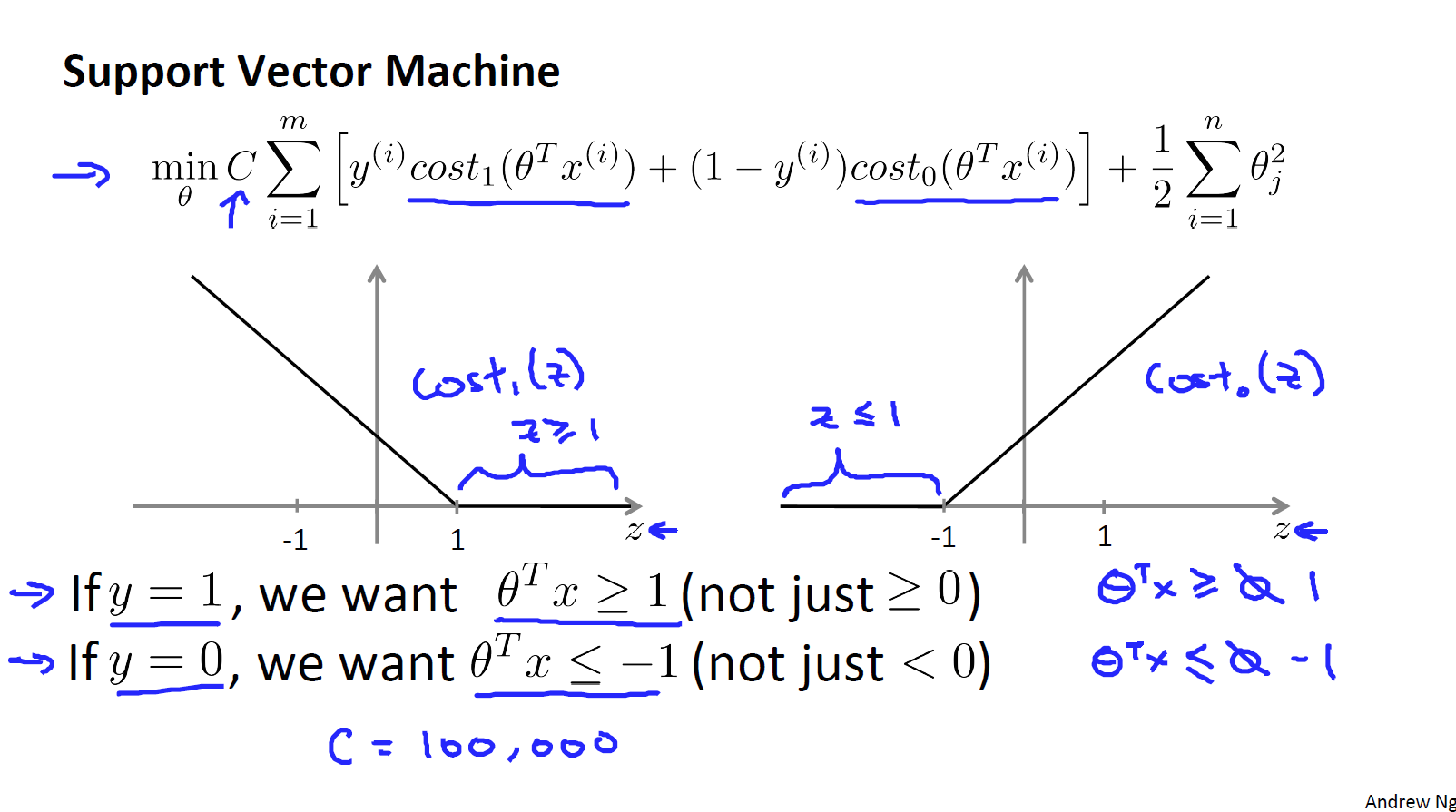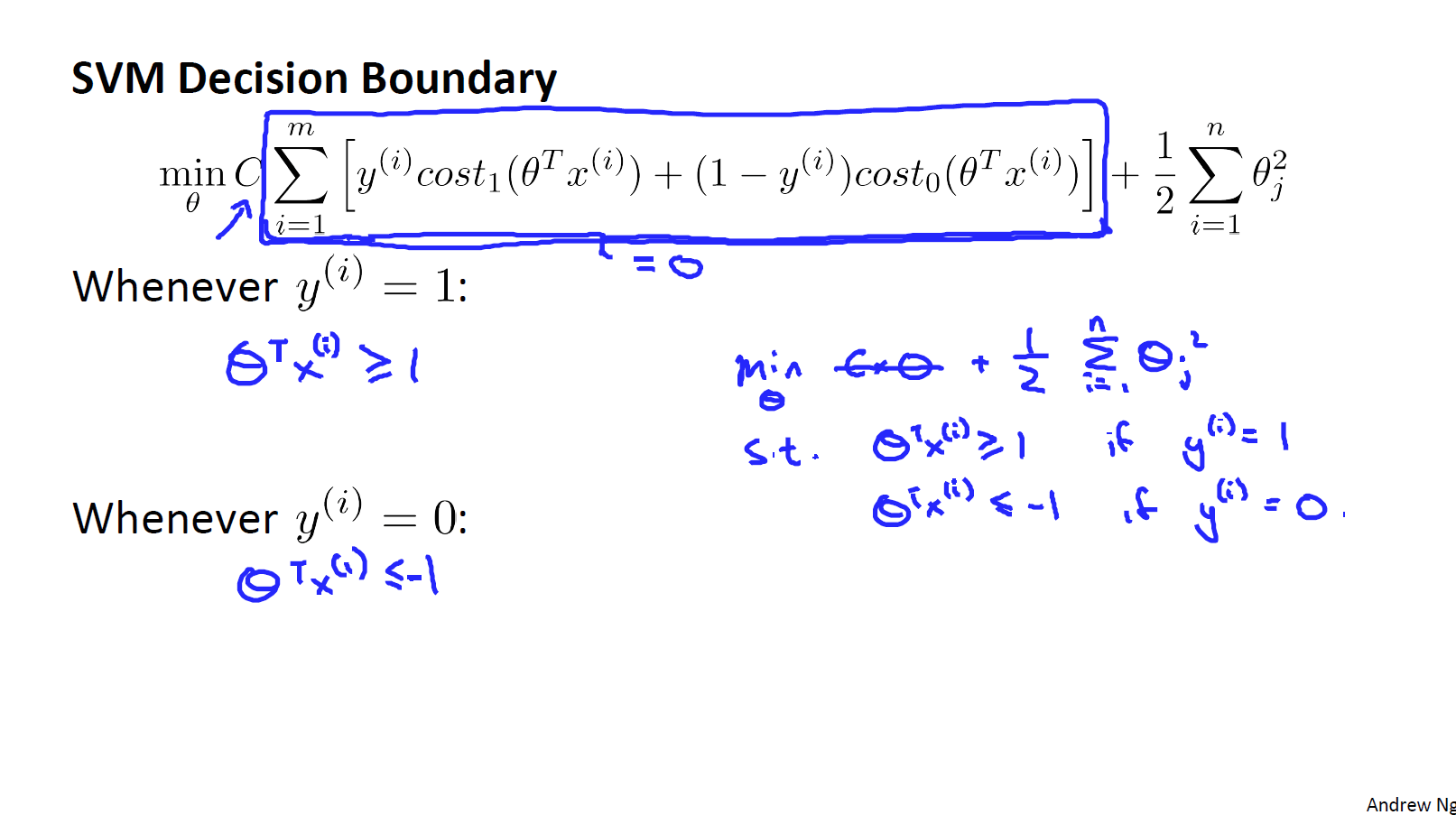y^(i) = 1，我们需要\theta^Tx^(i)>=1,同样对于一个样本y^(i) = 0，我们需要\theta^Tx^(i)<=-1.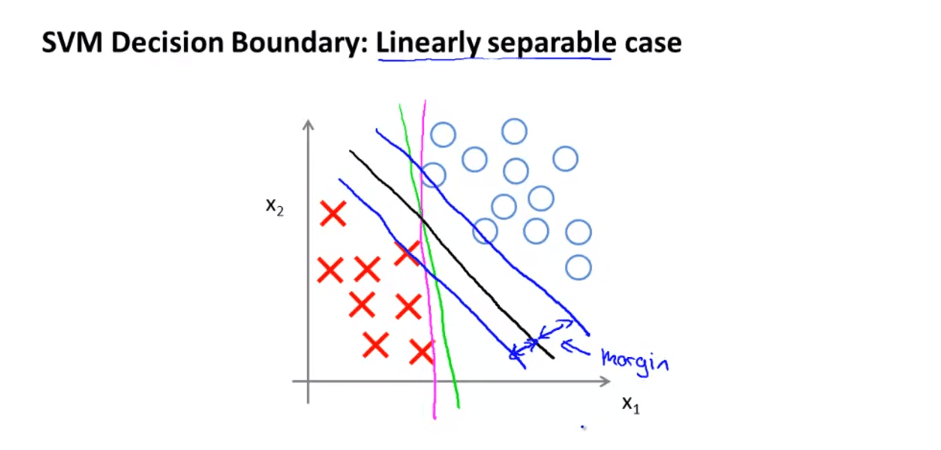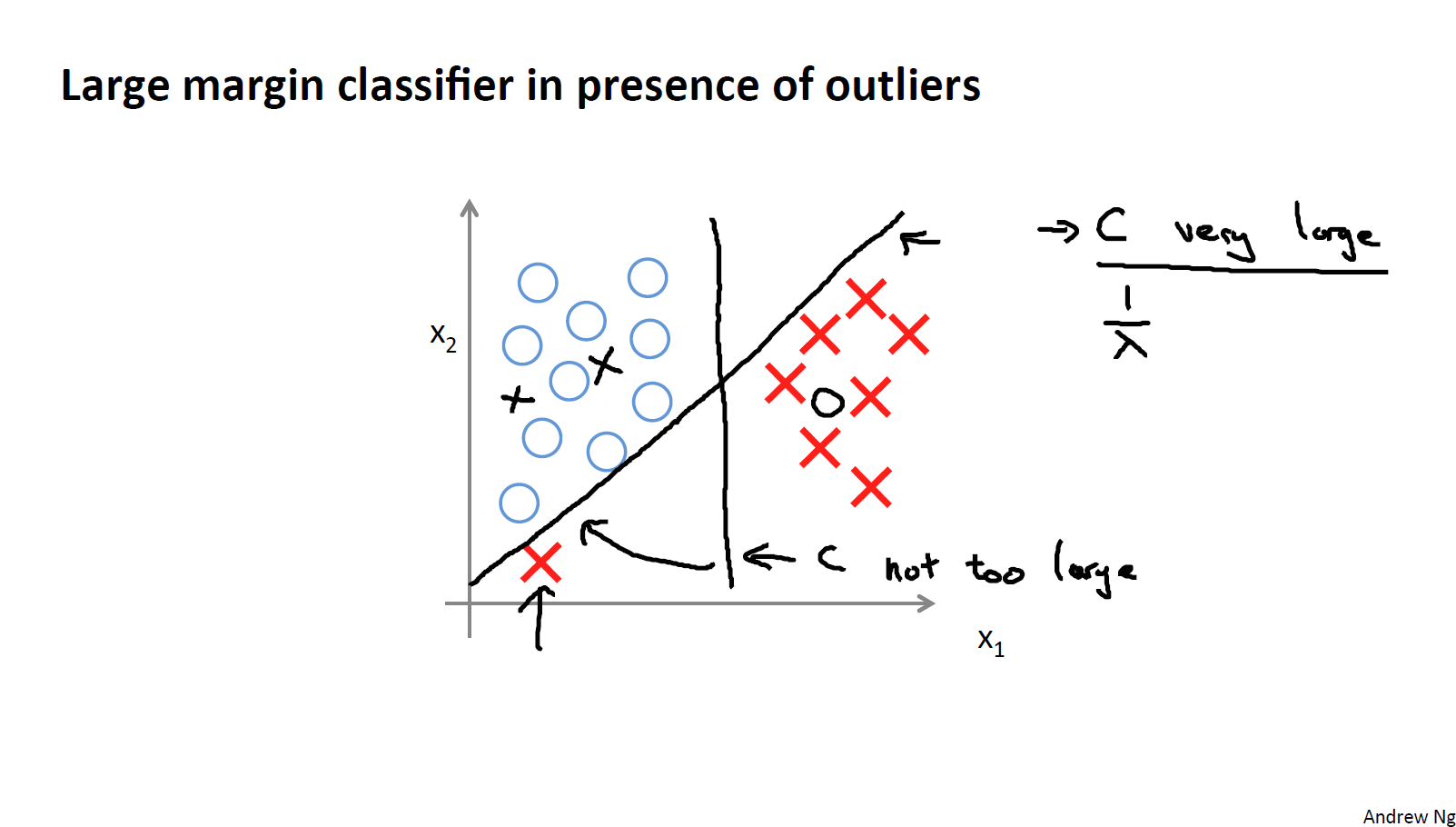## 核函数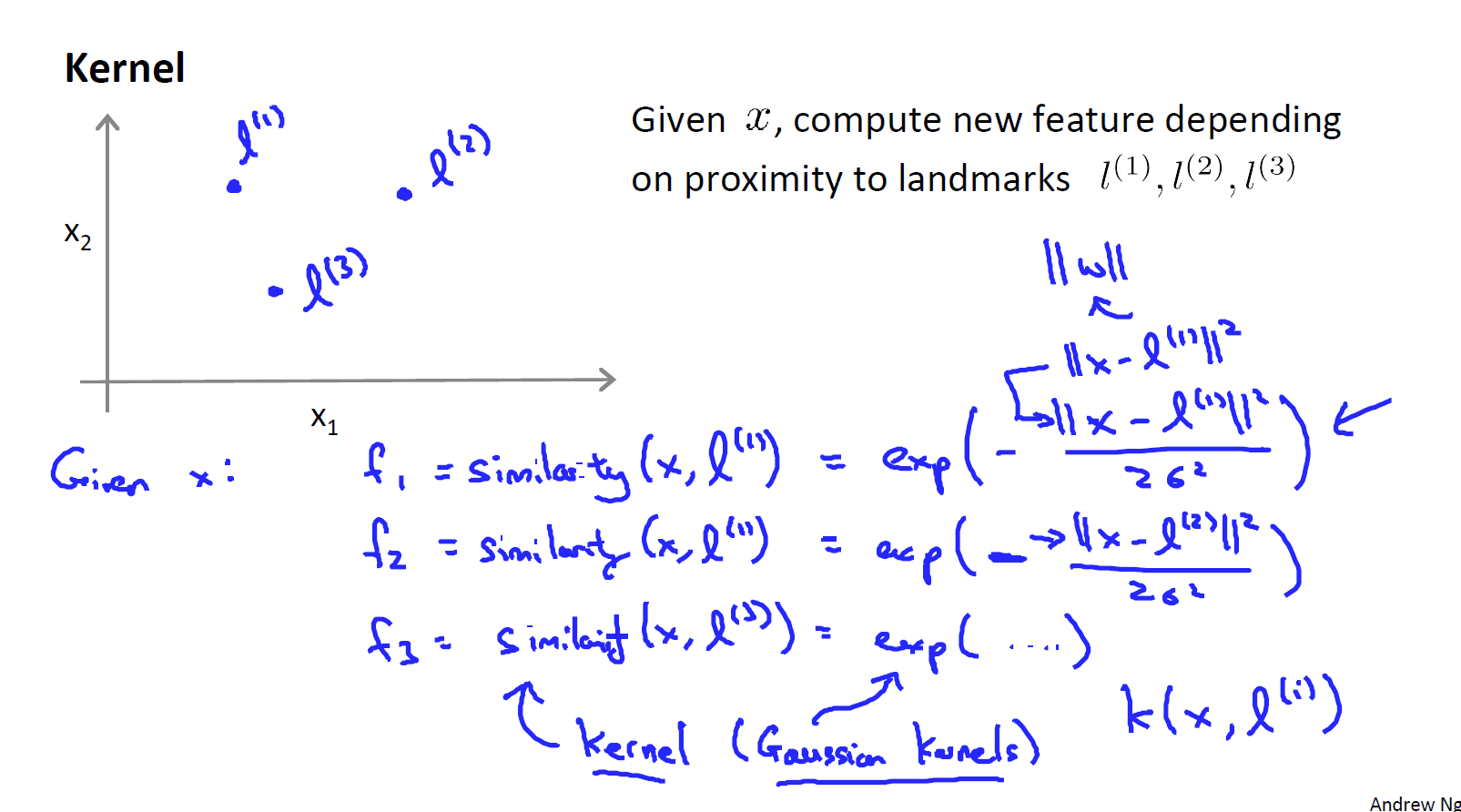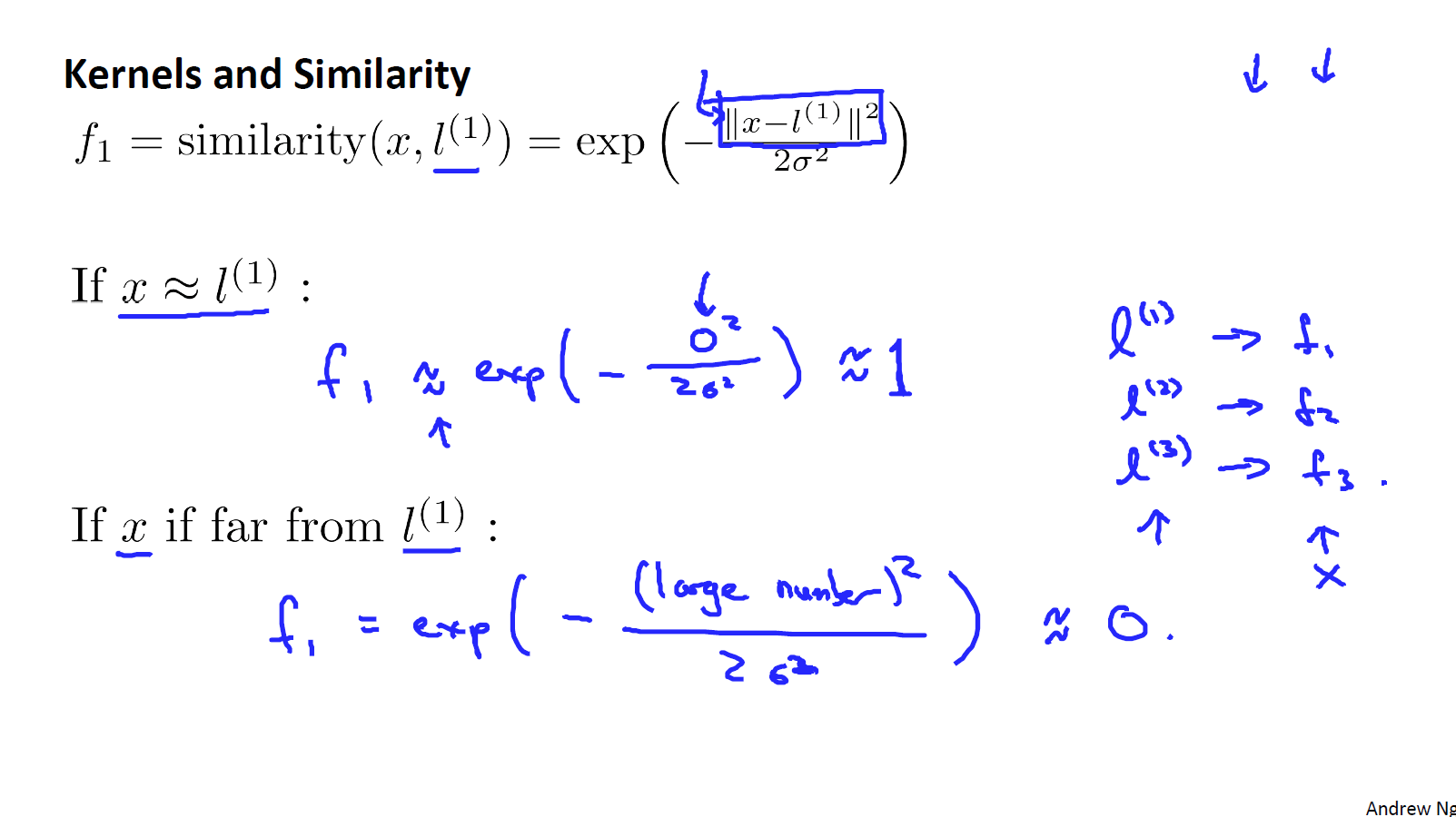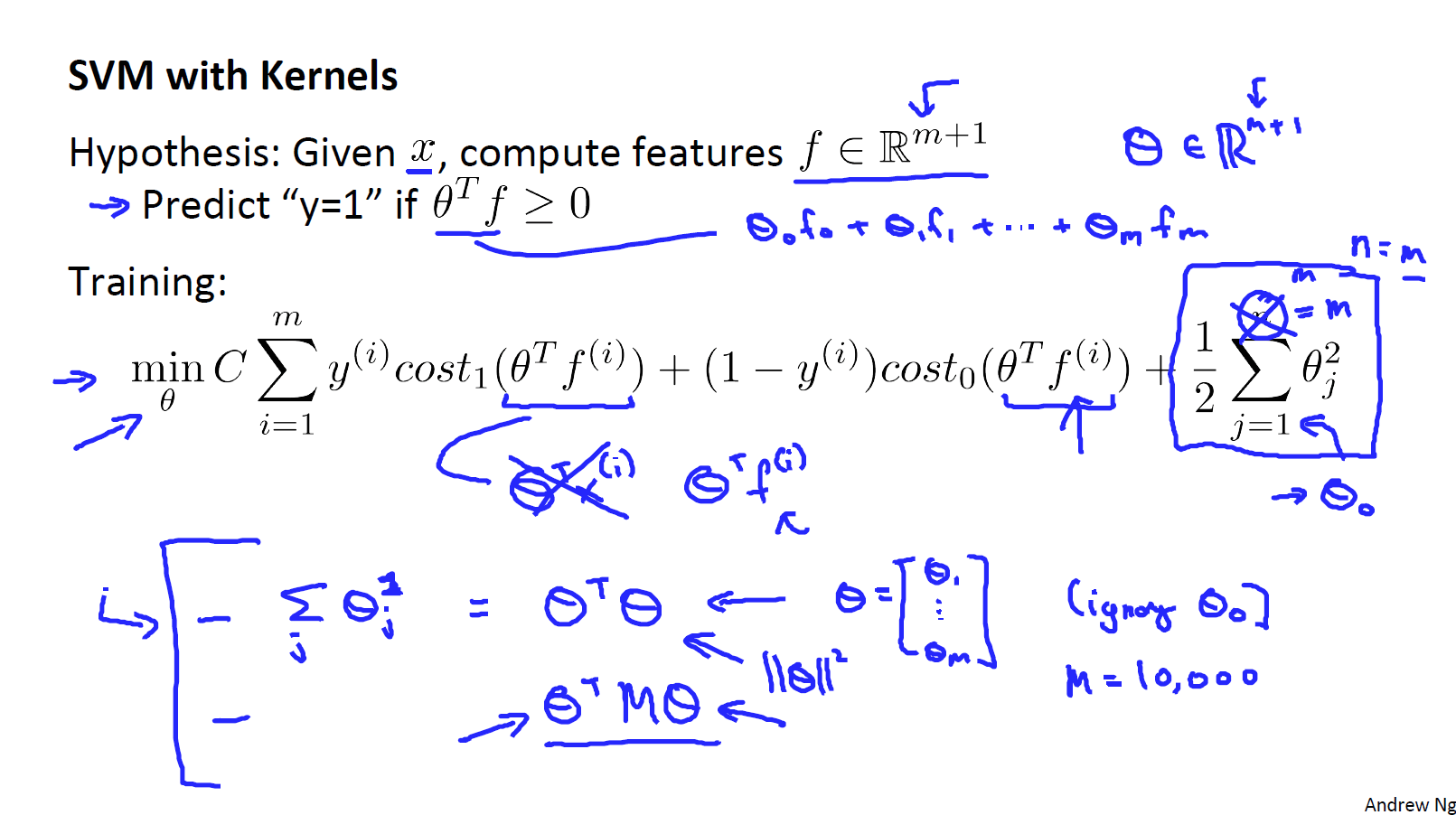C较大时，相当于 \lambda 较小，可能会导致过拟合，高方差；
C较小时，相当于\lambda较大，可能会导致低拟合，高偏差；
\sigma较大时，可能会导致低方差，高偏差；
\sigma较小时，可能会导致低偏差，高方差。## 实践SVM